## Example Questions

← Previous 1 3 4 5

### Example Question #1 : How To Find The Solution To An Inequality With Subtraction

Given that x = 2 and y = 4, how much less is the value of  2x2 –  2y than the value of  2y2 –  2x ?

12

52

2

28

28

Explanation:

First, we solve each expression by plugging in the given values for x and y:

2(22) – 2(4) = 8 – 8 = 0

2(42) – 2(2) = 32 – 4 = 28

Then we find the difference between the first and second expressions’ values:

28 – 0 = 28

### Example Question #2 : Inequalities

Solve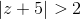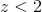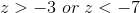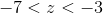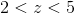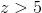Explanation:

Absolute value problems are broken into two inequalities: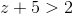and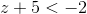.  Each inequality is solved separately to get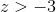and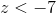.  Graphing each inequality shows that the correct answer is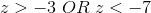.

### Example Question #3 : Inequalities

Which of the following inequalities defines the solution set to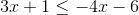?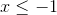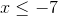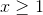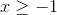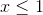Explanation:

First, move thes to one side.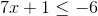Subtract by 1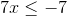Divide both sides by 7.### Example Question #10 : Inequalities

The cost, in cents, of manufacturingpencils is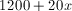. The pencils sell for 50 cents each. What number of pencils would need to be sold so that the revenue received is at least equal to the manufacturing cost?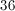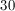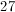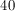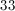Explanation:

If each pencil sells at 50 cents,pencils will sell at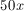. The smallest value ofsuch that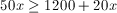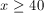### Example Question #4 : Inequalities

Solve the following inequality: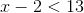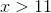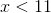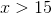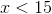Explanation:

To solve an inequality with subtraction, simply solve it is as an equation.

The goal is to isolate the variable on one side with all other constants on the other side. Perform the opposite operation to manipulate the inequality.

In this case add two to each side.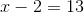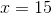### Example Question #5 : Inequalities

Solve the following inequality: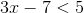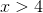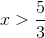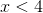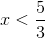Explanation:

To solve, simply treat it as an equation.

This means you want to isolate the variable on one side and move all other constants to the other side through opposite operation manipulation.

Remember, you only flip the inequality sign if you multiply or divide by a negative number.

Thus,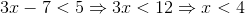### Example Question #6 : Inequalities

Solve |x – 5| ≤ 1

x ≤ 4 or x ≥ 6

-1 ≤ x ≤ 1

None of the answers are correct

0 ≤ x ≤ 1

4 ≤ x ≤ 6

4 ≤ x ≤ 6

Explanation:

Absolute values have two answers:  a positive one and a negative one.  Therefore,

-1 ≤ x – 5≤ 1 and solve by adding 5 to all sides to get 4 ≤ x ≤ 6.

### Example Question #1 : Inequalities

Solve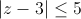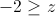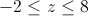All real numbers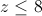No solutionsExplanation:

Absolute value is the distance from the origin and is always positive.

So we need to solve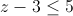and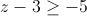which becomes a bounded solution.

Adding 3 to both sides of the inequality givesand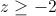or in simplified form### Example Question #8 : Inequalities

Given the inequality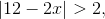which of the following is correct?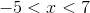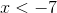or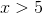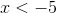or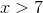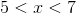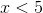ororExplanation:

First separate the inequality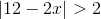into two equations.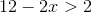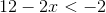Solve the first inequality.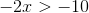Solve the second inequality.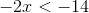Thus,or.

### Example Question #1 : Inequalities

What values of x make the following statement true?

|x – 3| < 9

–12 < x < 6

x < 12

6 < x < 12

–3 < x < 9

–6 < x < 12